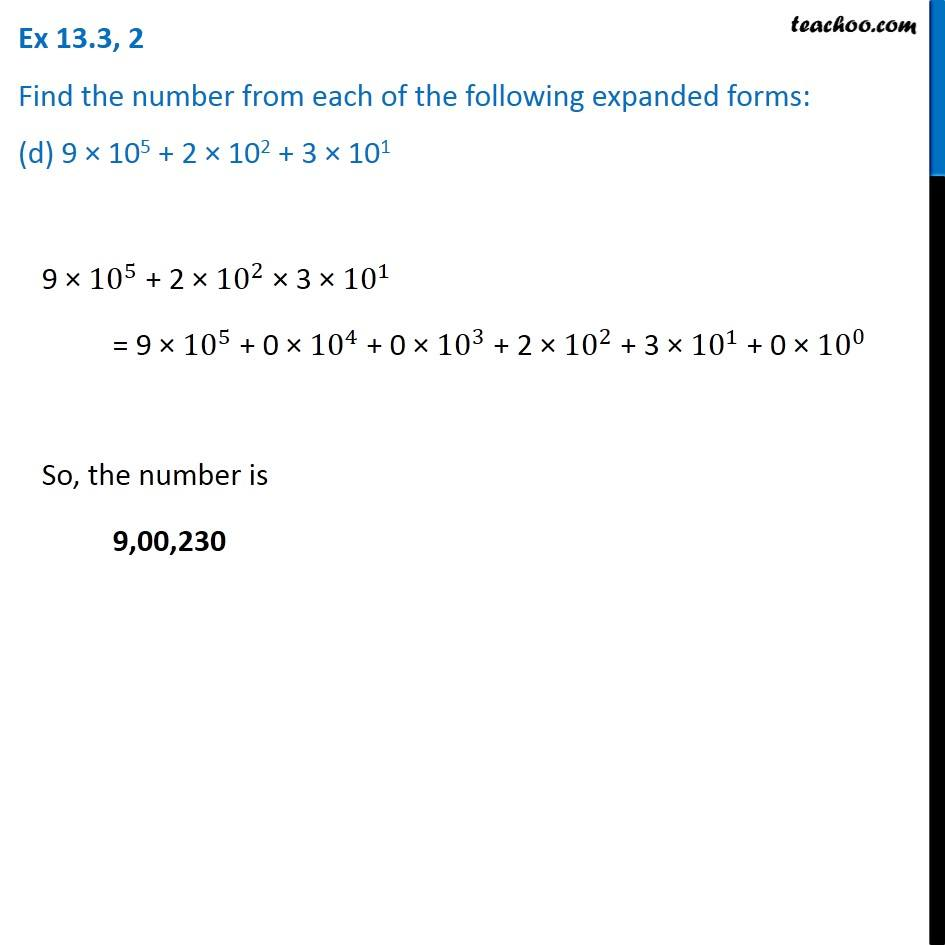1. Chapter 13 Class 7 Exponents and Powers
2. Serial order wise
3. Ex 13.3

Transcript

Ex 13.3, 2 Find the number from each of the following expanded forms: (d) 9 × 105 + 2 × 102 + 3 × 101 9 × 〖10〗^5 + 2 × 〖10〗^2 × 3 × 〖10〗^1 = 9 × 〖10〗^5 + 0 × 〖10〗^4 + 0 × 〖10〗^3 + 2 × 〖10〗^2 + 3 × 〖10〗^1 + 0 × 〖10〗^0 So, the number is 9,00,230